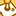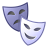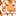# New to Qlik Sense

If you’re new to Qlik Sense, start with this Discussion Board and get up-to-speed quickly.

Announcements
Our May 2021 end-to-end product release from Data Integration to Data Analytics is out! READ DETAILS
cancel
Showing results for
Search instead for
Did you mean:Creator

## Create dimension from two dimensions

Hi All

I have two dimensions as follows:

Dimension1Dimension2
AA.1
AA.2
BB.1
BB.2

and I want to create the following dimensions

New dimensionsTotal
Asum where dimensions2 in (A.1,A.2)
Bsum where dimensions2 in (B.1,B.2)
Csum where dimensions2 in (A.2,B.2)

How can I create that?

Thanks in advance

Regards

11 RepliesPartner

Do you need to create the dimension with those parameters or perform the calculation defined by Dimension 1 = A and Dimension 2 = A.1?

In that case it could just be set analysis: Sum({<Dimension1 = {'A'}, Dimension2 = {'A.1'}>}Metric)

Sum({<Dimension1 = {'C'}, Dimension2 = {'A.1', 'B.2'}>}Metric)

To create a new dimension you can just use a conditional statement in the script:

if(Dimension2 = 'A.1', 'A',

if(Dimension2 = 'B.1', 'B',

if(Dimension2 = 'A2' OR Dimension2 = 'B.2', 'C'))) AS NewDimensionPartner

Using

a) Pick, WildMatch functions

b) Mapping table

```

Map_Table:
mapping Load * Inline [
Dimension2, NewDimension1
A.1, A
A.2, C
B.1, B
B.2, C
];

DataWithNewDimension:
LOAD
Dimension1,
Dimension2,
Pick(WildMatch(Dimension2, 'A.1', 'B.1','A.2', 'B.2'), 'A', 'B', 'C', 'C') AS NewDimension,
ApplyMap('Map_Table', Dimension2, 'Other') AS MapDimension

FROM [lib://mylink]
(html, utf8, embedded labels, table is @1);

Drop table Map_Table;
```Creator
Author

Thanks for your time mate. I made a mistake so I didn't explain well myself. Please see the original post

RegardsCreator
Author

Thanks for your time mate. I made a mistake so I didn't explain well myself. Please see the original post

RegardsCreator
Author

Anyonce who can help me?

Thanks

RegardsPartner

What is your original question/post?Creator
Author

I want to turn two columns in just one, like follows:

Dimension1Dimension2
AA.1
AA.2
BB.1
BB.2

into

New dimensionTotal
Asum where dimensions2 in (A.1,A.2)
Bsum where dimensions2 in (B.1,B.2)
Csum where dimensions2 in (A.2,B.2)Partner

In the script editor you can create that logic in the load statement of the table you are loading:

if(Dimension2 = 'A.1' OR Dimension2 = 'A.2', 'A',

if(Dimension2 = 'B.1' OR Dimension2 = 'B.2', 'B',

if(Dimension2 = 'A.2' OR Dimension2 = 'B.2', 'C'))) AS NewDimension

You can then use that dimension in the front end and sum(quanity) for that NewDimensionCreator
Author

Hello Joseph!

But in that case, wouldn't A.2 be only A instead of A and C?

RegardsTags